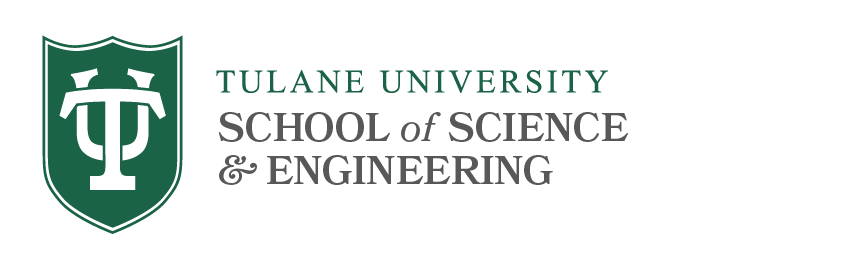# Algebra Qualifying Exams

## Syllabus Topics

### Elementary Number Theory (1)

Divisibility, prime numbers and the number of prime numbers. Unique factorization. Congruences, congruence theorems of Fermat and Euler. Euler's phi-function. Linear Diophantine equations, Pythagorean triples.

### Groups (3, 4)

Groups and important examples (quaternion group, Klein group, dihedral groups). Subgroups, cosets, Lagrange's theorem. Normal subgroups, factor groups. Homomorphisms, the homomorphism theorem, isomorphism theorem. Symmetric and alternating groups, permutation groups, Cayley's theorem. Simple groups. *Schreier's refinement theorem, *Jordan-Hölder theorem. Solvable groups. Automorphisms (inner and outer). Center, commutator subgroup. *Sylow theorems. Direct sums, *fundamental theorem on finitely generated abelian groups. Free groups, free abelian groups, presentations.

### Rings and Fields (3, 4)

Rings, ideals. Prime and maximal ideals in commutative rings. Field of quotients. Polynomial rings, matrix rings, boolean rings. Euclidean rings, PID's. Rings with chain conditions, Hilbert basis theorem. Prime fields. Algebraic and transcendental extensions. Algebraically closed fields. Finite fields. Galois groups, solvability of algebraic equations, constructions with straight edge and compass.

### Modules (2, 3, 4)

Exact sequences. Pullbacks and pushouts. Free, projective, injective modules. Projective, injective abelian groups. Homomorphism groups. Tensor products.

### Categories

Categories, important examples. Subobjects, kernels, cokernels, initial and terminal objects, limits, colimits. Functors, natural transformations. Adjoint functors, equivalence of categories, adjoint functor theorem.*

### Algebras (3)

Algebras, Group algebras, Quaternions, Finite-dimensional algebras, Frobenius theorem*, Tensor and exterior algebras*, Lie algebras.*

*no proofs required

References

1. A. Baker, Theory of Numbers, parts of Chapters 1, 2, 3, 8.
2. P. J. Hilton and U. Stammbach, A Course in Homological Algebra, parts of Chapters I-IV.
3. T. W. Hungerford, Algebra, parts of Chapters I-VI and X.
4. N. Jacobson, Basic Algebra I,II, parts of Chapters 1-4 in Volume I and Chapters 1, 3, 6, 8 in Volume II.